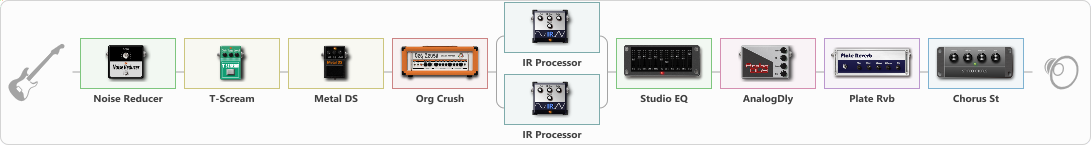# Disposable heroes

Discussion in 'ToneLib-GFX presets' started by Tony the Metalhead, Nov 12, 2021.

1. ### Tony the MetalheadActive Member

Disposable heroes

Preset name: Master Of Puppets

Effects chain:Effect: "Noise Reducer" (Dynamics / Filter), active - "yes"
{
"Sens" = 80
"Mode" = Hard
}

Effect: "T-Scream" (Overdrive / Distortion), active - "yes"
{
"Drive" = 5
"Tone" = 30
"Level" = 10
}

Effect: "Metal DS" (Overdrive / Distortion), active - "yes"
{
"Dist" = 60
"Bass" = 25
"Middle" = 40
"Treble" = 100
"Level" = 50
}

Effect: "Org Crush" (Amp simulators), active - "yes"
{
"Gain" = 60
"Bass" = 10
"Middle" = 25
"Treble" = 75
"Presence" = 85
"Master" = 25
"Level (dB)" = 6
}

Effect: "Splitter" (Dynamics / Filter)
{
"A-Bypass" = Off
"A-Pan" = 0
"A-Level" = 55
"B-Bypass" = Off
"B-Pan" = 0
"B-Level" = 55

'A' branch:
{

Effect: "IR Processor" (Cabinets), active - "yes"
{
"IR" = disposable_hero_IR
"Low Cut (Hz)" = 175
"Hi Cut (kHz)" = 20.0
"Mix" = 100
"Level (dB)" = 0
}
}
'B' branch:
{

Effect: "IR Processor" (Cabinets), active - "yes"
{
"IR" = disposable_hero_IR
"Low Cut (Hz)" = 175
"Hi Cut (kHz)" = 20.0
"Mix" = 100
"Level (dB)" = 0
}
}
}

Effect: "Studio EQ" (Dynamics / Filter), active - "yes"
{
"31 Hz" = -4
"62 Hz" = -5
"125 Hz" = -1
"250 Hz" = -7
"500 Hz" = -6
"1 kHz" = -10
"2 kHz" = -8
"4 kHz" = -8
"8 kHz" = -10
"16 kHz" = 10
"Level (dB)" = 6
}

Effect: "AnalogDly" (Delay), active - "yes"
{
"Time" = 25
"Feedback" = 25
"Tone" = 50
"Mix" = 25
}

Effect: "Plate Rvb" (Reverberation), active - "yes"
{
"Time" = 1.8
"PreDelay" = 5
"LoDamp" = 25
"HiDamp" = 65
"Mix" = 25
}

Effect: "Chorus St" (Modulation / Sfx), active - "yes"
{
"Speed" = 1.8
"Depth" = 5
"Center" = 2.0
"Mix" = 25
}

Note: You will need to download and install the ToneLib-GFX software to use the preset.

File size:
2 KB
Views:
2,533